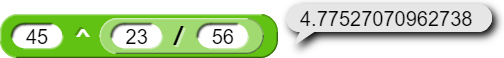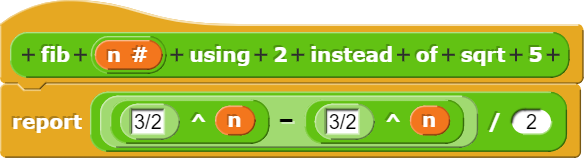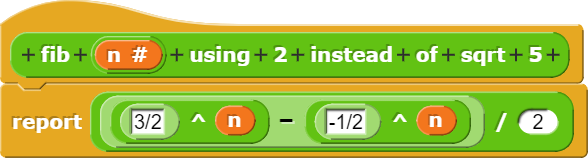# Keep Fractional Exponents Exact in Bignums

I.e.and

should be returning true, andshould be returning a x/y like

does.

I had this problem trying to use (sqrt 5).

Regardless of the error in BigNum (monadic sqrt should return fn.sqrt)

reportMonadic: function (fname, n) {
...
case 'sqrt':
return sqrt(n);


it's implemented this way in SchemeNumber.js as described in Revised^6 Report on the Algorithmic Language Scheme
sqrt

The sqrt procedure may return an inexact result even when given an exact argument.

(sqrt -5)
‌‌⇒ 0.0+2.23606797749979i ; approximately

^ power operator

(expt z1 z2)‌‌procedure
...
For an exact real number object z1 and an exact integer object z2, (expt z1 z2) must return an exact result. For all other values of z1 and z2 , (expt z1 z2) may return an inexact result, even when both z1 and z2 are exact.

Well that's stupid.

Shouldn't that return function (num) {return sqrt(num);} or something like that?

Snap/libraries/bignums.js

reportMonadic: function (fname, n) {
...
case 'sqrt':
return fn.sqrt(n);


Unfortunately not corrected even in v7.

@warped_wart_wars, the square root of five is irrational! There's no way to return an exact value.

And similarly, it's only for integer exponents that they guarantee exactness.

What we could do is build in a symbolic algebra system, in which expressions count as values, and so (5 ^ (1/2)) would report (5 ^ (1/2)). That's what Al Cuoco wants. I don't think we're going to do that, but SICP includes the beginnings of symbolic expressions as data if you're interested.

Not quite sure what you mean...
But now is

case 'sqrt':
return sqrt(n);


Should be

case 'sqrt':
return fn.sqrt(n);


Ah. Thanks. I'll fix it.

Yes, there is! "$$\sqrt5$$" is how to represent the square root of 5 exactly.

Specifically in Binet's Formula. Without this fixed, I get things like

which should be 2.

Pardon me. There is no way to return an exact sqrt(5) in the number representations understood by the computer hardware.

Someone was claiming that all the sqrt(5) calls cancel out when you do the algebra. You should try using, you know, 2, or 183, or something, in place of all the sqrt(5) calls and see what you get.

Are those just random numbers you chose?

Oh and

Oh and I don't think the denominators all being powers of 2 is a coincidence.

Yes, but they still have an effect on the resulting number.

Well, 2 is an integer very near sqrt(5), but 183 was just what my fingers hit. (So probably not uniformly distributed. :~) )

I guess the result of your experiment is that sqrt(5) does not disappear from the formula, but it might effectively disappear by being multiplied by itself on every appearance, or something.(3/2 is the simplified form of (/ (+ 1 2) 2))

That can't be right; you're subtracting two identical expressions, so the answer will always be zero.

Oh yeah, right. The second is supposed to be (/ (- 1 2) 2) which simplifies to $$-(1/2)$$.

Edit: Here's the right formula:Click on yellow Alonzo top left then click on button on right that says new topic

Sorry, I meant that when finding integer Fibonacci numbers, all the $$\sqrt{5}$$ somehow cancel, since you get an integer result.

P.S. I just learned you can do LaTex math inside double dollar signs.

This topic was automatically closed 30 days after the last reply. New replies are no longer allowed.